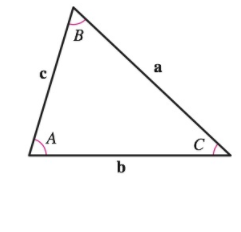Chapter 11, Problem 1PS

Chapter
Section
Textbook Problem

# ProofUsing vectors, prove the Law of Sines: If a, b, and c are the three sides of the triangle shown in the figure, then sin A ‖ a ‖ = sin B ‖ b ‖ = sin C ‖ c ‖ .To determine

To prove: For given three sides of the triangle are a, b, and c, prove Law of sines by vector method.

sinAa=sinBb=sinCc

Explanation

Given:

The three sides of the triangle are a, b, and c.

Formula used:

If θ is the angle between two nonzero vectors u and v, where 0θπ, then

u×v=uvsinθ

Proof:

Formula of angle between two vectors is

b×a=basinCc×a=casinBc×b=cbsinA

Further let

b=c+a, a=bc and c=ba.

put b=c+a in c×b:

c×b=c×(c+a)=c×c+c×a=c×a</

### Still sussing out bartleby?

Check out a sample textbook solution.

See a sample solution

#### The Solution to Your Study Problems

Bartleby provides explanations to thousands of textbook problems written by our experts, many with advanced degrees!

Get Started

#### Convert the expressions in Exercises 8596 radical form. x4/3

Finite Mathematics and Applied Calculus (MindTap Course List)

#### In Exercises 13-20, sketch a set of coordinate axes and plot each point. 16. (3, 4)

Applied Calculus for the Managerial, Life, and Social Sciences: A Brief Approach

#### 45 15 63 127

Study Guide for Stewart's Multivariable Calculus, 8th